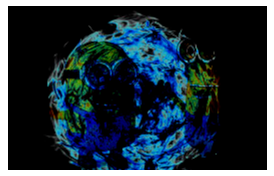Open in App
Not now

# Python PIL | ImageChops.subtract() and ImageChops.subtract_modulo() method

• Last Updated : 27 Jun, 2019

PIL is the Python Imaging Library which provides the python interpreter with image editing capabilities.

## ImageChops.subtract() method –

This method subtracts two images, dividing the result by scale and adding the offset. If omitted, scale defaults to 1.0, and offset to 0.0.

Syntax: ImageChops.subtract(image1, image2, scale = 1.0, offset = 0)

Parameters:
image1: first image
image2: second image
scale: it is floating point value (if the value keeps on increasing, resultant image becomes darker and darker)
offset: numeric value (if value keeps on increasing, resultant image becomes lighter)

Return Value: An Image

 `# This will import Image and ImageChops modules``from` `PIL ``import` `Image, ImageChops`` ` `# Opening Images``im ``=` `Image.``open``(r``"C:\Users\Admin\Pictures\images.png"``)``im2 ``=` `Image.``open``(r``"C:\Users\Admin\Pictures\download.PNG"``)`` ` `# subtracting im and im2``im3 ``=` `ImageChops.subtract(image1 ``=` `im, image2 ``=` `im2)`` ` `# showing resultant image``im3.show()`

Output:## ImageChops.subtract_modulo() method –

This method is also used to subtract two images but without clipping the result.

Syntax: ImageChops.subtract_modulo(image1, image2)

Parameters: This method takes two images as parameters.

Return Value: An Image.

 `# This will import Image and ImageChops modules``from` `PIL ``import` `Image, ImageChops`` ` `# Opening Images``im ``=` `Image.``open``(r``"C:\Users\Admin\Pictures\images.png"``)``im2 ``=` `Image.``open``(r``"C:\Users\Admin\Pictures\download.PNG"``)`` ` `# subtracting im and im2``im3 ``=` `ImageChops.subtract_modulo(image1 ``=` `im, image2 ``=` `im2)`` ` `# showing resultant image``im3.show()`

Output:My Personal Notes arrow_drop_up